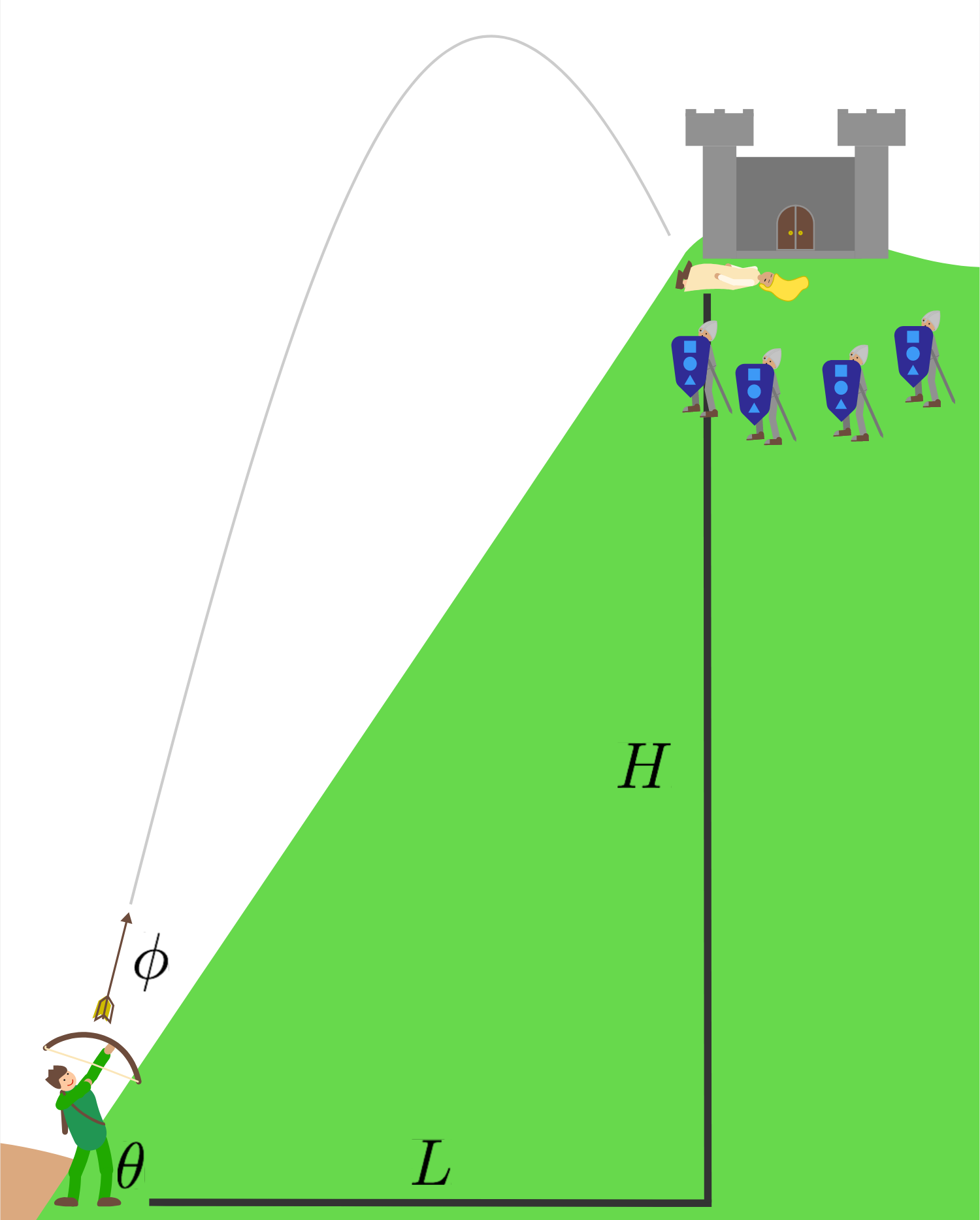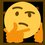# Projectile on a Slope$$\gamma=\theta +\phi$$

$\ddot { x } =0\\ \dot { x } ={ v }_{ 0 }\cos { \gamma } \\ x={ (v }_{ 0 }\cos { \gamma } )t\\ x\overset { set }{ = } L\\ { v }_{ 0 }\cos { \gamma } t=s\cos { \theta } \\ \Rightarrow t=\frac { s\cos { \theta } }{ { v }_{ 0 }\cos { \gamma } } \\ \ddot { y } =g\\ \dot { y } =gt+{ v }_{ 0 }\sin { \gamma } \\ y=\frac { g }{ 2 } { t }^{ 2 }+({ v }_{ 0 }\sin { \gamma } )t\\ y\overset { set }{ = } H\\ \Rightarrow s\sin { \theta } =\frac { g }{ 2 } { t }^{ 2 }+{ (v }_{ 0 }\sin { \gamma } )t$

\begin{aligned} \Rightarrow s\sin { \theta } & = \frac { g }{ 2 } { (\frac { s\cos { \theta } }{ { v }_{ 0 }\cos { \gamma } } ) }^{ 2 }+({ v }_{ 0 }\sin { \gamma } )(\frac { s\cos { \theta } }{ { v }_{ 0 }\cos { \gamma } } ) \\ s\sin { \theta } & = (\frac { g{ s }^{ 2 }\cos ^{ 2 }{ \theta } }{ 2{ { v }_{ 0 } }^{ 2 } } )\frac { \sin ^{ 2 }{ \gamma } +\cos ^{ 2 }{ \gamma } }{ \cos ^{ 2 }{ \gamma } } +(s\cos { \theta } )\tan { \gamma } \\ (\frac { g{ s }^{ 2 }\cos ^{ 2 }{ \theta } }{ 2{ { v }_{ 0 } }^{ 2 } } )\tan ^{ 2 }{ \gamma +(s\cos { \theta } )\tan { \gamma } +(\frac { g{ s }^{ 2 }\cos ^{ 2 }{ \theta } }{ 2{ { v }_{ 0 } }^{ 2 } } -s\sin { \theta ) } } & = 0 \\ \tan { \gamma } & = \frac { -(s\cos { \theta } )\pm \sqrt { { (s\cos { \theta } ) }^{ 2 }-4(\frac { g{ s }^{ 2 }\cos ^{ 2 }{ \theta } }{ 2{ { v }_{ 0 } }^{ 2 } } )(\frac { g{ s }^{ 2 }\cos ^{ 2 }{ \theta } }{ 2{ { v }_{ 0 } }^{ 2 } } -s\sin { \theta ) } } }{ 2(\frac { g{ s }^{ 2 }\cos ^{ 2 }{ \theta } }{ 2{ { v }_{ 0 } }^{ 2 } } ) } \\ \gamma \left( g,s,{ v }_{ 0 },\theta \right) & = \boxed { \tan ^{ -1 }{ \frac { \pm \sqrt { { { { { { v }_{ 0 } }^{ 4 } }-g }^{ 2 }{ s }^{ 2 }\cos ^{ 2 }{ \theta } +{ { v }_{ 0 } }^{ 2 }2g{ s }\sin { \theta } } } -{ { v }_{ 0 } }^{ 2 } }{ g{ s }\cos { \theta } } } } \end{aligned}Note by Gandoff Tan
2 years, 1 month ago

This discussion board is a place to discuss our Daily Challenges and the math and science related to those challenges. Explanations are more than just a solution — they should explain the steps and thinking strategies that you used to obtain the solution. Comments should further the discussion of math and science.

When posting on Brilliant:

• Use the emojis to react to an explanation, whether you're congratulating a job well done , or just really confused .
• Ask specific questions about the challenge or the steps in somebody's explanation. Well-posed questions can add a lot to the discussion, but posting "I don't understand!" doesn't help anyone.
• Try to contribute something new to the discussion, whether it is an extension, generalization or other idea related to the challenge.
• Stay on topic — we're all here to learn more about math and science, not to hear about your favorite get-rich-quick scheme or current world events.

MarkdownAppears as
*italics* or _italics_ italics
**bold** or __bold__ bold
- bulleted- list
• bulleted
• list
1. numbered2. list
1. numbered
2. list
Note: you must add a full line of space before and after lists for them to show up correctly
paragraph 1paragraph 2

paragraph 1

paragraph 2

[example link](https://brilliant.org)example link
> This is a quote
This is a quote
    # I indented these lines
# 4 spaces, and now they show
# up as a code block.

print "hello world"
# I indented these lines
# 4 spaces, and now they show
# up as a code block.

print "hello world"
MathAppears as
Remember to wrap math in $$ ... $$ or $ ... $ to ensure proper formatting.
2 \times 3 $2 \times 3$
2^{34} $2^{34}$
a_{i-1} $a_{i-1}$
\frac{2}{3} $\frac{2}{3}$
\sqrt{2} $\sqrt{2}$
\sum_{i=1}^3 $\sum_{i=1}^3$
\sin \theta $\sin \theta$
\boxed{123} $\boxed{123}$

## Comments

Sort by:

Top Newest

This would be a good problem for the physics Community section

- 2 years ago

Log in to reply

It's from the classical mechanics course.

- 2 years ago

Log in to reply

×

Problem Loading...

Note Loading...

Set Loading...